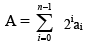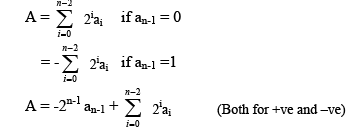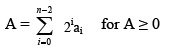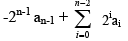# Integer Representation Computer Science Engineering (CSE) Notes | EduRev

## Computer Science Engineering (CSE) : Integer Representation Computer Science Engineering (CSE) Notes | EduRev

The document Integer Representation Computer Science Engineering (CSE) Notes | EduRev is a part of the Computer Science Engineering (CSE) Course Computer Architecture and Organisation (CAO) - Notes & all.
All you need of Computer Science Engineering (CSE) at this link: Computer Science Engineering (CSE)

Integer Representation
An eight bit word can be represented the numbers from zero to 255 including

00000000 = 0
00000001 = 1
- - - - - - -
11111111 = 255
In general if an n-bit sequence of binary digits an-1, an-2 …..a1, a0; is interpreted as unsigned integer A.Sign magnitude representation:
There are several alternative convention used to represent negative as well as positive integers, all of which involves treating the most significant (left most) bit in the word as sign bit. If the sign bit is 0, the number is +ve and if the sign bit is 1, the number is –ve. In n bit word the right most n-1 bit hold the magnitude of integer.
For an example,
+18 = 00010010
- 18 = 10010010 (sign magnitude)
The general case can be expressed as follows:There are several drawbacks to sign-magnitude representation. One is that addition or subtraction requires consideration of both signs of number and their relative magnitude to carry out the required operation. Another drawback is that there are two representation of zero. For an example:
+010 = 00000000
-010 = 10000000 this is inconvenient.

2’s complement representation:
Like sign magnitude representation, 2’s complement representation uses the most significant bit as sign bit making it easy to test whether the integer is negative or positive. It differs from the use of sign magnitude representation in the way that the other bits are interpreted. For negation, take the Boolean complement (1’s complement) of each bit of corresponding positive number, and then add one to the resulting bit pattern viewed as unsigned integer. Consider n bit integer A in 2’s complement representation. If A is +ve then the sign bit an-1 is zero. The remaining bits represent the magnitude of the number.The number zero is identified as +ve and therefore has zero sign bit and magnitude of all 0’s. We can see that the range of +ve integer that may be represented is from 0 (all the magnitude bits are zero) through 2n-1-1 (all of the magnitude bits are 1).
Now for –ve number integer A, the sign bit an-1 is 1. The range of –ve integer that can be represented is from -1 to -2n-1

2’s complement, A =Defines the twos complement of representation of both positive and negative number.
For an example:
+18 = 00010010
1’s complement = 11101101
2’s complement = 11101110 = -18

Offer running on EduRev: Apply code STAYHOME200 to get INR 200 off on our premium plan EduRev Infinity!

## Computer Architecture and Organisation (CAO) - Notes & all

66 videos|72 docs|39 tests

,

,

,

,

,

,

,

,

,

,

,

,

,

,

,

,

,

,

,

,

,

;